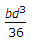# Mechanical Engineering - Engineering Mechanics

### Exercise :: Engineering Mechanics - Section 3

1.

If the masses of both the bodies, as shown in the below figure, are doubled, then the acceleration in the string will beA. same B. half C. double

Explanation:

No answer description available for this question. Let us discuss.

2.

The loss of kinetic energy during inelastic impact, is given by(where m1 = Mass of the first body,m2 = Mass of the second body, and u1 and u2 = Velocities of the first and second bodies respectively.)

 A.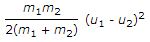B.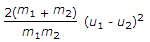C.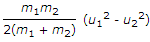D.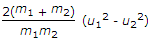Explanation:

No answer description available for this question. Let us discuss.

3.

The centre of gravity of a hemisphere lies at a distance of 3r / 8 from its base measured along the vertical radius.

 A. Correct B. Incorrect

Explanation:

No answer description available for this question. Let us discuss.

4.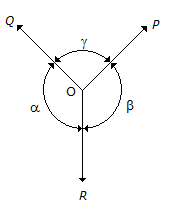The above figure shows the three coplaner forces P, Q and R acting at a point O. If these forces are in equilibrium, then

 A.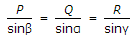B.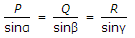C.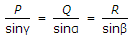D.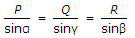Explanation:

No answer description available for this question. Let us discuss.

5.

Moment of inertia of a rectangular section having width (b) and depth (d) about an axis passing through its C.G. and parallel to the depth (d), is

 A.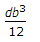B.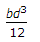C.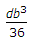D.